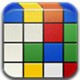# One of these things is not like the others

Here’s a puzzle I came across a few days ago (thanks, Mom!):

Which of the following does not belong?

1. Large green square
2. Large red circle
3. Large green circle
4. Small green circle

The source seems to be Marilyn vos Savant’s Ask Marilyn column in Parade, January 19, 2014, according to this forum and this newsletter and some other sources.

The answer that’s given is 3. 1 is the only square, 2 is the only red, 4 is the only small – so 3 is the only one that’s not the only somethi9ng (that is, it shares its size, its color, and its shape with at least one other of each option).

The natural question for me became: are there other similar puzzles? We can think of this puzzle as picking out four points on a cube. The three dimensions are large/small, green/red, and circle/square. So the original puzzle, abstracted away, looks something like this:

(original version picture goes here)

The four filled-in circles represent the four shapes named in the puzzle. The one that “does not belong” is the one that’s adjacent to the other three, indicated in blue.

Now, let’s consider how many ways there are to pick four vertices of a cube. There are${8 \choose 4} = 70$ ways to do that, but how many of those are essentially different? I find six, illustrated below:The top left one represents the puzzle we were given. But in each of the others there’s no way to distinguish one of the four vertices from the other three. Here they are, in turn:This is the original puzzle. There are eight ways to pick our four vertices that look like this one – pick the blue point (the central one, which will turn out to be distinguished from the rest) to be any of the eight vertices.This is the “tetrahedral” version. The four chosen vertices form a tetrahedron, so there’s no way to distinguish them from each other; there are two ways to do this.The four chosen vertices form a rectangle, and are all indistinguishable. There are six such rectangles (each of the twelve edges of the cube is in exactly one, and there are two edges of the cube in each rectangle)The four chosen vertices form a square face of the cube. The vertices are all indistinguishable. There are six of them.You can play connect-the-dots with this “twisted” arrangement; there are two central vertices (blue) and two outer vertices (orange), but there’s no way to distinguish between the two blues or between the two oranges. There are 24 such arrangements – pick the central edge (connecting the blues) and then there are two ways to complete.This “three-on-one” arrangement is the most complicated case. Three vertices form an L (on the bottom of the cube), and the two outer ones (black) can be distinguished from the middle one (orange). The blue one is the one outside of the L. There are twenty-four such arrangements – they’re determined by the L, which is determined by choosing a face (six ways) and a vertex within that face (four ways).

But this doesn’t make a good puzzle: out of a large red circle, a large red square, a large green square, and a small green circle, which one is distinct? You could argue for the small green circle (it’s the only small, corresponding to the blue vertex) but there’s a more convoluted argument for the large red square (of the three larges, it’s the “central” one).

These six families exhaust all the possible ways to pick four vertices from a cube – their sizes add up to 8 + 2 + 6 + 6 + 24 + 24 = 70. (This is the number of orbits of a certain group action and should follow from the Polya enumeration theorem but it was easier to just work these out individually.)

Of these, I believe the puzzle as given is the best one, but this is a matter of taste.

## 4 thoughts on “One of these things is not like the others”

1.Ralph says:

In the context of Hardy’s anecdote about Ramanujan and 1729, I know I’ve heard a joke “paradox” – what is the smallest number that has no interesting properties whatsoever?

2.Jonathan Kariv says:

Suppose one step up you could ask find the odd one out:
(1,1,1,1)
(1,1,1,0)
(1,1,0,1)
(1,0,1,1)
(0,1,1,1)
Or the 6 option 5-D example.

3.Maurizio Epifani says:

Reblogged this on Ai margini.

4.asipos says:

I used this trick once successfully to get the right answer to a multiple-choice question in a chemistry contest. (I figured that every wrong answer had to be one step beside the correct one.)